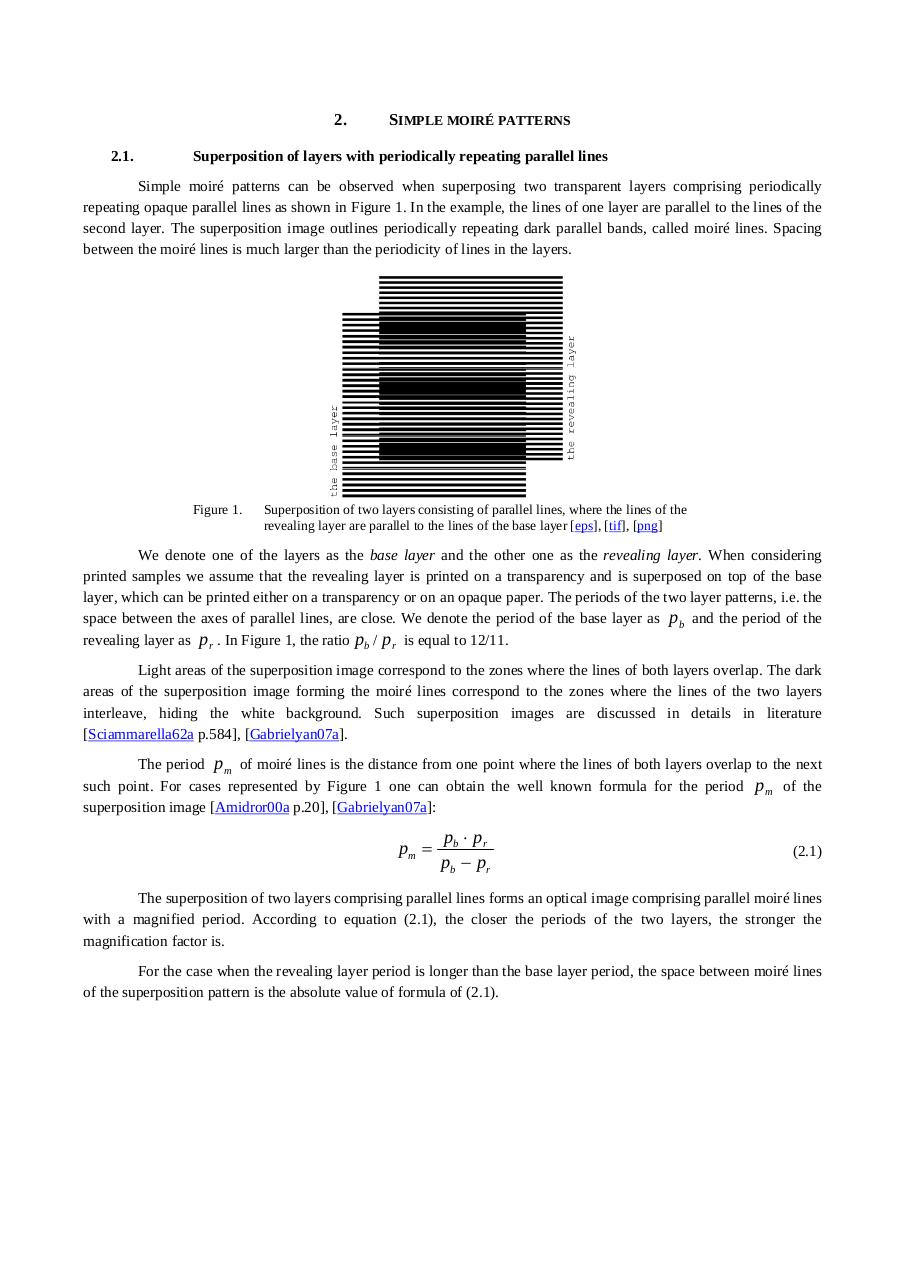# 10.1.1.399.1946.pdfPage 1 2 34524

#### Text preview

2.
2.1.

SIMPLE MOIRÉ PATTERNS

Superposition of layers with periodically repeating parallel lines

Simple moiré patterns can be observed when superposing two transparent layers comprising periodically
repeating opaque parallel lines as shown in Figure 1. In the example, the lines of one layer are parallel to the lines of the
second layer. The superposition image outlines periodically repeating dark parallel bands, called moiré lines. Spacing
between the moiré lines is much larger than the periodicity of lines in the layers.

Figure 1.

Superposition of two layers consisting of parallel lines, where the lines of the
revealing layer are parallel to the lines of the base layer [eps], [tif], [png]

We denote one of the layers as the base layer and the other one as the revealing layer. When considering
printed samples we assume that the revealing layer is printed on a transparency and is superposed on top of the base
layer, which can be printed either on a transparency or on an opaque paper. The periods of the two layer patterns, i.e. the
space between the axes of parallel lines, are close. We denote the period of the base layer as pb and the period of the
revealing layer as pr . In Figure 1, the ratio pb / pr is equal to 12/11.
Light areas of the superposition image correspond to the zones where the lines of both layers overlap. The dark
areas of the superposition image forming the moiré lines correspond to the zones where the lines of the two layers
interleave, hiding the white background. Such superposition images are discussed in details in literature
[Sciammarella62a p.584], [Gabrielyan07a].
The period pm of moiré lines is the distance from one point where the lines of both layers overlap to the next
such point. For cases represented by Figure 1 one can obtain the well known formula for the period pm of the
superposition image [Amidror00a p.20], [Gabrielyan07a]:

pm =

pb ⋅ pr
pb − p r

(2.1)

The superposition of two layers comprising parallel lines forms an optical image comprising parallel moiré lines
with a magnified period. According to equation (2.1), the closer the periods of the two layers, the stronger the
magnification factor is.
For the case when the revealing layer period is longer than the base layer period, the space between moiré lines
of the superposition pattern is the absolute value of formula of (2.1).